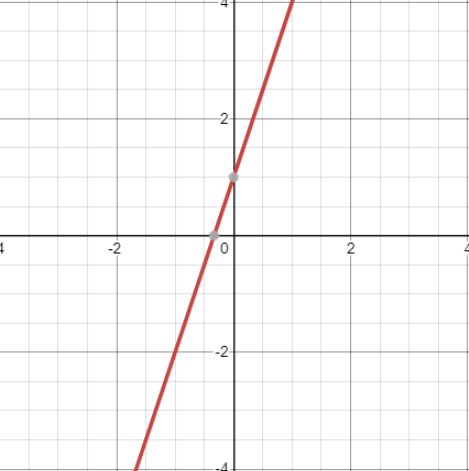Ask question

# Item 11 Graph the linear equation y=3x+1 .# Item 11 Graph the linear equation y=3x+1 .

Question
Linear equations and graphsasked 2020-12-28
Item 11 Graph the linear equation y=3x+1 .

## Answers (1)2020-12-29

Since the equation is the form of y=mx+b, it is considered to be a linear equation. And the result of the graph will be a straight line. In graphing linear equation, assume that the value of the x-coordinate to be 0 to get the first point in the form (0,y) and assume y-coordinate to be 0 to get the second point in the form (x,0)
Assuming x=0, y=3x+1
y=3(0)+1
y=1 The first point will be (0,1) Assuming y=0, y=3x+1
0=3x+1
$$\displaystyle{x}=-\frac{{1}}{{3}}\ {\quad\text{or}\quad}\ {x}=-{0.333333}$$
And the second point will be $$\displaystyle{\left(-\frac{{1}}{{3}},{0}\right)}$$
Plotting the two points (0,1) and $$\displaystyle{\left(-\frac{{1}}{{3}},{0}\right)}$$ will result in### Relevant Questionsasked 2020-12-03

A graph of a linear equation passes through ( -2,0) and (0,-6) is the $$3x-y=6$$, both ordered pairs solutions for the equationasked 2020-12-06
Given linear equation y = -3x
a. find the y-intercept and slope.
b. determine whether the line slopes upward, slopes downward, or is horizontal, without graphing the equation.
c. use two points to graph the equation.asked 2021-01-19
Given linear equation y = -1+2x
a. find the y-intercept and slope.
b. determine whether the line slopes upward, slopes downward, or is horizontal, without graphing the equation.
c. use two points to graph the equation.asked 2020-11-29
Given linear equation y = 1.5x
a. find the y-intercept and slope.
b. determine whether the line slopes upward, slopes downward, or is horizontal, without graphing the equation.
c. use two points to graph the equation.asked 2020-11-02
Given linear equation y = 3+4x
a. find the y-intercept and slope.
b. determine whether the line slopes upward, slopes downward, or is horizontal, without graphing the equation.
c. use two points to graph the equation.asked 2020-11-05
Given linear equation y = -0.75x-5
a. find the y-intercept and slope.
b. determine whether the line slopes upward, slopes downward, or is horizontal, without graphing the equation.
c. use two points to graph the equation.asked 2021-01-04
Given linear equation y = 6 − 7x
a. find the y-intercept and slope.
b. determine whether the line slopes upward, slopes downward, or is horizontal, without graphing the equation.
c. use two points to graph the equation.asked 2020-12-21
Given linear equation y = -8-4x
a. find the y-intercept and slope.
b. determine whether the line slopes upward, slopes downward, or is horizontal, without graphing the equation.
c. use two points to graph the equation.asked 2021-02-25
Given linear equation y = -3
a. find the y-intercept and slope.
b. determine whether the line slopes upward, slopes downward, or is horizontal, without graphing the equation.
c.use two points to graph the equationasked 2020-11-05
Given linear equation y = 0.5x – 2
a. find the y-intercept and slope.
b. determine whether the line slopes upward, slopes downward, or is horizontal, without graphing the equation.
c. use two points to graph the equation.
...